“No one shall expel us from the paradise which Cantor has created for us.” — Hilbert on Cantor’s creation of Set Theory

### Update

There is now a Flashcard deck on Quizlet for Set Theory:

### Starting out

Set theory is a notation used to describe sets. A set is a list of objects where repetition does not matter and order does not matter.

To denote a set, use curly braces. To denote the set of all good British tea you could write something like Tea = {PG tips, Tetley, Yorkshire Tea, Twinnings}.

If you like PG tips a lot you could rewrite that as tea = {PG tips, PG tips, PG tips} which would result it in just equaling tea = {PG tips} as repetition does not matter.

What if we want to denote a set of all the bad British tea? That’s easy. You would do this, tea = {} or tea = which is the symbol for the empty set.

You can create sets within sets for example you could have a set of British teas with good British teas in it. Tea = { {PG Tips, PG tips black}, {Tetly} }.

Two sets are equal when the contents are equal. For example, {2, 3, 3} is equal to {2, 3} but {{2}} is not equal to {2}.

You can create infinite sets using mathematics. For example, to create a set of all the positive even numbers one could make the set:

X = {y | y is all the positive even numbers}

You do not have to write out all the positive even numbers, just show that it’s implied by using a pipe character, “|”, to show the mathematical formula being applied to the set.

Any mathematical formula can be applied here. For example to produce a set of all positive integers between 0 and 100 one can do this:

X = {s | 0 < s < 100}

All sets are denoted with a capital letter.

#### Performing operations on sets

A subset is a set that exists entirely in the parent or original set. For example, given the set x = {1, 2, 3, 4} a subset may be y = {1, 2} or q = {4}.

A subset contains any elements that exist within the parent set. Just to reiterate x = {Tetley, PG Tips} is a subset of Tea = {PG tips, Tetley, Yorkshire Tea, Twinnings}.

The notation for a subset is the symbol ⊆. To say that x is a subset of Tea one would say x ⊆ Tea.

In other words, ⊆ X where X is any set at all.

A bit vector is a notation used to describe the placement of elements within a subset. Let’s say we have a set, x = {1, 2, 3, 4, 5, 6, 7, 8, 9} and we have a subset y = {1, 3, 6} we could denote y using bit vectors as:

Y = (1, 0, 1, 0, 0 , 1, 0, 0 , 0)

This represents that y is a subset of x with elements 1, 3, 6. If the order of a set mattered, this would also be a handy notation to represent where the elements are in both lists.

Two sets are equal if the first set is a subset of the second set and the second set is a subset of the first step, essentially they have the same elements. In mathematical terms this is:

A ⊆ B and B ⊆ A then A = B

### Union of 2 sets

The union of 2 sets is a set containing all the elements of the first set and all the elements of the second set.

The notation to describe this is a union of A and B is A ∪ B.

The mathematical formula for this is the union of sets is A ∪ B = {x | x ∈ A or x ∈ B}

Again, anything that is both in A and B will appear in the set which is a union of the 2 sets.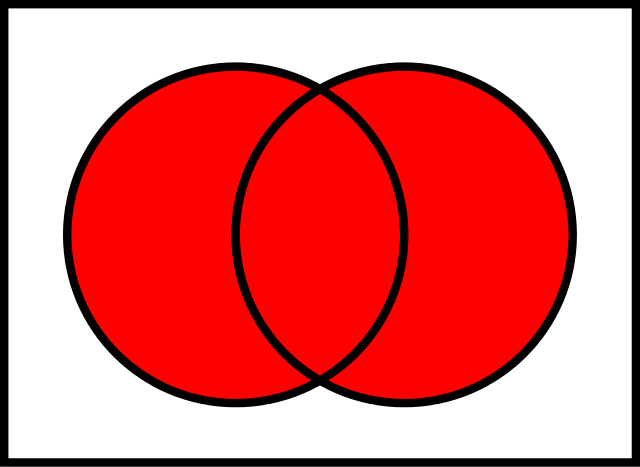The union of 2 sets whereby everything found in both sets is added together. By Watchduck (a.k.a. Tilman Piesk) — Own work, Public Domain, https://commons.wikimedia.org/w/index.php?curid=11149747

The union of A (where A is any set) and (empty set) is just A as does not have any elements to add to the set.

The union of A = {1, 2} and B = {3, 4} is A ∪ B = {1, 2, 3, 4]

Let’s try to create the union of 2 sets in bit notation.

C = (1, 0, 0, 0, 1) and B = (1, 1, 0, 1, 1)

The union of C and B (C ∪ B) is {1, 1, 0, 1, 1}

### Intersection of 2 sets

The intersection of 2 sets is a subset which contains only the items found in both A and B. The mathematical symbol for this is ∩. The intersection of A and B is A ∩ B.

If A contains the elements {1, 2, 3} and B contains the element {2} then A ∩ B (the intersection of A and B) is the subset {2} since 2 exists in both of these sets.

The intersection of any set, A with the empty set is just the empty set because the empty set does not have any elements. A ∩ =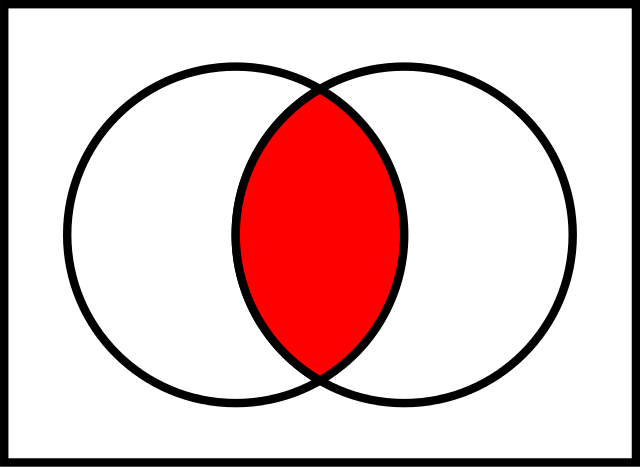The intersection of 2 sets. The red part represents the intersection. By Watchduck (a.k.a. Tilman Piesk) — Own work, Public Domain, https://commons.wikimedia.org/w/index.php?curid=11149747

The mathematical notation for the intersection is A ∩ B = {x : x ∈ A and X ∈ B}.

If A is a subset of B, A ⊆ B then A ∩ B.

Intersection of 2 sets represented using bit vectors.

Let C = (1, 0, 0, 0 ,1) and D = (1, 1, 0, 0, 1 ) then C ∩ D is equal to

C ∩ D = (1, 0, 0, 0, 1)

### The Relative Complement

The relative compliment is the set of A ∪ B minus B. In other words, it is all the numbers in set A that are not in set B.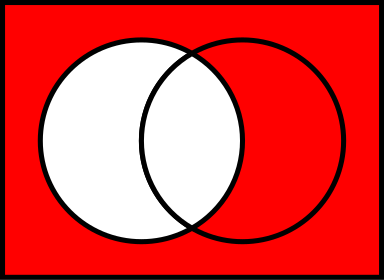By Watchduck (a.k.a. Tilman Piesk) — Own work, Public Domain, https://commons.wikimedia.org/w/index.php?curid=11149747

Another way we can look at this is that if A is a set then the relative compliement of A is everything that is not in A.

The mathematical notation for the relative compliment is the “ \” symbol. To denote A as a relative compliment of B one would say A \ B.

The mathematical formula for the relative compliment is A \ B = {X | X ∈ A and ∉ B}

As an example, suppose there exists a set A = {4, 7, 8} and there also exists a set B = {4, 9, 10} then A \ B = {7, 8}

A \ = A for any set A because the empty set has nothing to takeaway.

Another good example is this:

A = {1, 2, 3} and B = the natural numbers then A \ B results in the empty set, ∅. This is because when removing B from A we are not adding anything, we cannot add anything to the original set A.

Let’s try this with Bit Vectors.

Set C = (1, 0, 0, 0, 1) and D = (1, 1, 0, 0, 1) then C \ D = (0, 0, 0, 0, 0)

### The Complement

There exists a set within set theory called Universal set, denoted as U. The universal set is the set of every single object.

The complement is the universal set take away a given set.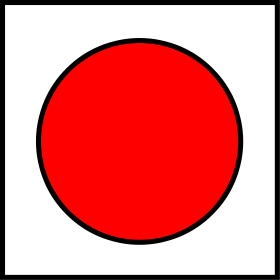By Watchduck (a.k.a. Tilman Piesk) — Own work, Public Domain, https://commons.wikimedia.org/w/index.php?curid=11149747

When given a set, denoted by the red circle we can find the compliment of this set by removing it from the universal set; the white square.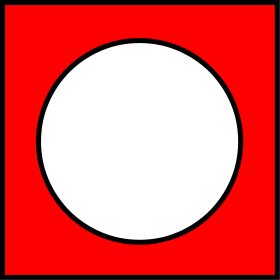By Watchduck (a.k.a. Tilman Piesk) — Own work, Public Domain, https://commons.wikimedia.org/w/index.php?curid=11149747

The above image represents the universal set where the previous red circle is taken away, thus the compliment of A where A is the red circle is shown above.

The mathematical notation for the complement is A^c or ~ a.

The mathematical formula for the complement is:

~A = {x ∉ A} = U — a

The complement of set C, representred by the bit vector (1, 0, 0, 0, 1) which is (0, 1, 1, 1, 0). Essentially reversing the bit vector.

The compliment of the universal set is the universal set take away the universal set which is ∅.

Some readers may be more familar with calling the compliment “not”. Not A is the set of everything that is not A.

### The Symetric Difference

The Symmetric Difference of two sets is everything that is in set A and everything that is in set B but not in both excluding the universal set.

The mathematical formula for this is:

a∆b = {x | (a ∈ aand x ∉ b) or (x ∉ a and x ∈ b)}

In short, this is the union of 2 sets minus the common elements.

Here’s an example: A = {4, 7, 8} and B = {4, 9, 10} so A ∆ B = {7, 8, 9, 10}

### The Algebra of Sets

#### Communiative law

The communicative law states we can swap numbers over and still get the same result.

We can use algebra within set theory such as the communicative law. In algebra, a * b = b * a and a + b = b + a. Therefore the union of 2 sets A ∪ B = B ∪ A.

#### Assiocative Law

The assiocative law states that it doesn’t matter how we group the numbers.

Suppose that A, B, C, U are sets with A⊆U, B⊆U, C⊆U then:

A union of (B ∪ c) = (a ∪b) ∪ c, a ∩b (b ∩ c) = a ∩ b ∩ c

In algebra this is simply

a+(b+c) = (a+b)+c or a * (b*c) = (a*b)*c

#### Distributive law

The distributive law states that the number a in the equation a(b+c) = ab+ac is that a is seperately applied to each term of the equation b + c resulting in ab + ac.

In simpleton terms, this law shows that the result of first adding several numbers together and then multiplying the final sum by some number is the same as first multiplying each term seperately by the number and then adding the products.

A ∪ (B ∩ C) = (A ∪ B) ∩ (A ∪ C)

The union of A with (B intersection C) is the intersection of of (a union b) and (a union c).

### Demorgan’s Laws

Demorgan’s laws are a set of transformative rules created by Augustus De Morgan, many people write De Morgan’s Laws as Demorgan’s laws so that notation is used here.

Note: The lecturers at the University of Liverpool use “~” to mean compliment.

One of demorgans law says that if you take the union of two sets the compliment of that is the same as taking the compliment of A and the compliment of B and finding the intersection of A and B.

In set theory notation:

~(A ∪ B) = ~A ∩ ~B

The second law states that the compliment of the intersection is the union of the compliment of those 2 sets

~(A ∩ B) = ~A ∪ ~ B

Below is an image showing Demorgan’s laws applied to two different venn diagrams. Note: the line above the letters denotes the compliment.

### Cardinality

The cardinality is how many set elements are in a set. Lets say S = {1, 2, 3, 3} then |S| is equal to 3 since repition does not matter.

Let’s say S = { {a}, {a, b}, {d}, e }.. The cardinality of S, |S| is 4 because there exists 4 elements at the top level.

What is |N| where N is the natural numbers? Infinity. Or in set theory, aleph-zero.

|R| where r is the real numbers is aleph-1.

If you want to learn more about Aleph’s and how to count past infinity, watch this video.

If A and B are sets then |A| + |B| = |A ∪ B|

If A and B are sets then |A ∪ B| = |A| + |B| — |A ∩ B|

### Naive Set Theory

This is called naive because it suffers from paradoxs.

For example:

A barber is the man who shaves all those, and only those, men who do not shave themselves.

Who shaves the barber?

We can write this in set theory. Note: => means therefore.

Let B be the barber. If B shaves himself then B ∈ {those who shaved by B}=> B ∈ {those who don’t shave themselves}=> B does not shave himself.

If B does not shave himself then B ∈ {those who don’t shave themselves} => B ∈ {those shaved by B}=> B shaves himself.

This is a paradox. In a more general case, this is called Russel’s Paradox.

Russels paradox shows that the object {x | P(x) } is not always meaningful. Below is Russel’s paradox shown symbolically.

Let X= {R | R ∉ R}, then X ∈ X < = > R ∉ R.

### Example questions

Use these for revision purposes. Answers at the end.

1. Suppose there are 100 third-year students. 40 of them take the module “Sequential Algorithms” and 80 of them take the module “Multi-Agent Systems”. 25 of them took both modules. How many students took neither?
2. Is Ø ∈ Ø?
3. Is Ø ⊆ {5}?
4. What is the result of {x : x ∈ N, 5 < x < 6}? (Note: N is set of all natural numbers)
5. What numbers are held in the set A = {x | x ∈ N, X > 1 and X < 10}?
6. Evaluate U — Ø

### Symbols you need to memorise

Medium doesn’t allow tables so this looks awkward. For a better and more complete table, go here.